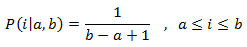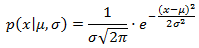# Random Numbers with C++

### Basic Concepts

We are going to use C++11 and more specifically the header random which introduces random number generation facilities.

This library allows to produce random numbers using combinations of generators and distributions:

• Generators: Objects that generate uniformly distributed numbers.
• Distributions: Objects that transform sequences of numbers generated by a generator into sequences of numbers that follow a specific random variable distribution, such as Uniform, Normal or Binomial.

Distribution objects generate random numbers by means of their operator() member, which takes a generator object as argument:

``````std::default_random_engine generator;
std::uniform_int_distribution<int> distribution(1,6);
int dice_roll = distribution(generator);  // generates number in the range 1..6``````

All standard generators defined in the library are random number engines, which are a kind of generators that use a particular algorithm to generate series of pseudo-random numbers. These algorithms need a seed as a source of randomness, and this seed can either be a single value or an object with a very specific generate() member function (see seed_seq for more info). A typical source of randomness for trivial tasks is time, such as the information provided by time or system_clock::now.

### Generators/ engines

They use an algorithm to generate pseudo-random numbers based on an initial seed:

Particular instantiations of generator engines:

• default_random_engineIt is the library implemention's selection of a generator that provides at least acceptable engine behavior for relatively casual, inexpert, and/or lightweight use.
• minstd_randA simple multiplicative congruential pseudo-random number generator.
``x = x * 48271 % 2147483647``
• mt19937A Mersenne Twister pseudo-random generator of 32-bit numbers with a state size of 19937 bits.
```typedef mersenne_twister_engine<uint_fast32_t,
32,624,397,31,0x9908b0df,11,0xffffffff,7,0x9d2c5680,15,0xefc60000,18,1812433253>
mt19937;```
• mt19937_64A Mersenne Twister pseudo-random generator of 64-bit numbers with a state size of 19937 bits.
```typedef mersenne_twister_engine<uint_fast64_t,
64,312,156,31,0xb5026f5aa96619e9,
29,0x5555555555555555,
17,0x71d67fffeda60000,
37,0xfff7eee000000000,
43,6364136223846793005> mt19937_64;```

### Distributions

Popular ones:

• uniform_int_distribution: Probability density function (pdf)  ->• uniform_real_distribution: Probability density function (pdf)  ->• normal_distribution: Probability density function (pdf)  ->** For more information on C++ random library check this website & for more general info on random numbers check here.

### True Random Number Generators?

Traditionally, computers rely on mathematical algorithms to generate ‘random’ numbers. These type of random numbers are called pseudorandom numbers. For a finite sample, good pseudorandom number generators will also reproduce statistics that are consistent with true randomness.

But all pseudorandom number generators rely on a seed to generate the random sequences. This means that anybody who has access to the seed will be able to generate the same sequence of random numbers.

Moreover, most pseudorandom numbers have a finite period. Good pseudorandom number generators (e.g. Mersenne Twister MT19937) have huge periods. But eventually, if we wait long enough, the sequence will repeat itself.

** Check this website for more info on true random numbers and a method to produce them from quantum phenomena.

### Example Codes

An example of generating random numbers using the default engine of C++ and a uniform distribution.

`// To compile:// g++ -std=c++11 uniform_int_distribution.cpp // uniform_int_distribution#include <iostream>#include <string>#include <random> int main(){  const int nrolls = 10000; // number of experiments  const int nstars = 95;    // maximum number of stars to distribute   int seed = 6;  std::default_random_engine generator(seed);  std::uniform_int_distribution<int> distribution(0,9);   int p={};   std::cout << "Without changing the seed:" << "\n";  for (int i=0; i<10; ++i) {    std::cout << distribution(generator) << " " ;  }  std::cout << "\n";   for (int i=0; i<nrolls; ++i) {    int number = distribution(generator);    ++p[number];  }   std::cout << "uniform_int_distribution (0,9):" << std::endl;  for (int i=0; i<10; ++i)    std::cout << i << ": " << std::string(p[i]*nstars/nrolls,'*') << std::endl;   return 0;}`

An example of generating random numbers using various engines and a normal distribution.

`// To compile:// g++ -std=c++11 normal_distribution.cpp // normal_distribution#include <iostream>#include <string>#include <random> int main(){  const int nrolls=10000;  // number of experiments  const int nstars=100;    // maximum number of stars to distribute   //std::mt19937 generator;  //std::minstd_rand generator;  std::default_random_engine generator;  std::normal_distribution<double> distribution(5.0,2.0);   int p={};   for (int i=0; i<nrolls; ++i) {    double number = distribution(generator);    if ((number>=0.0)&&(number<10.0)) ++p[int(number)];  }   std::cout << "normal_distribution (5.0,2.0):" << std::endl;   for (int i=0; i<10; ++i) {    std::cout << i << "-" << (i+1) << ": ";    std::cout << std::string(p[i]*nstars/nrolls,'*') << std::endl;  }   return 0;}`

Remark:  In the previous examples, the seed is not changing, i.e., the produced random numbers are the same at every execution of the codes.

Below, by changing the seed we manage to produce different sequences at every execution.

`// To compile:// g++ -std=c++11 seed.cpp #include <iostream>#include <chrono>#include <random> int main(){  // construct a trivial random generator engine from a time-based seed:  unsigned int seed = std::chrono::system_clock::now().time_since_epoch().count();  std::default_random_engine generator(seed);   std::normal_distribution<double> distribution (0.0,1.0);   std::cout << "some Normal-distributed(0.0,1.0) results:" << std::endl;  for (int i=0; i<10; ++i)    std::cout << distribution(generator) << " ";  std::cout << std::endl;   return 0;}`

## How to test the quality of a sequence of random numbers?

The test summarized below can be used for both true or pseudo random numbers.